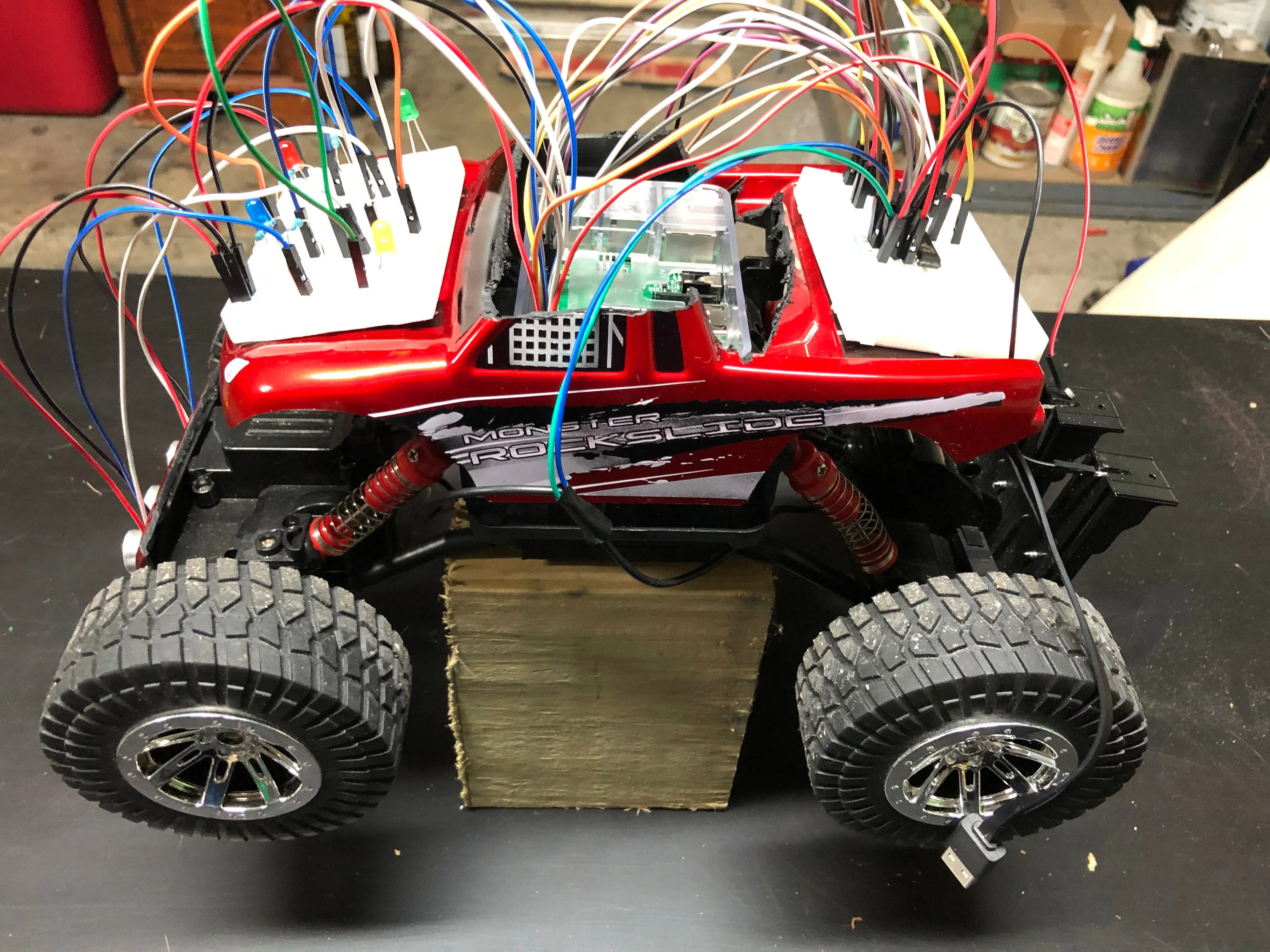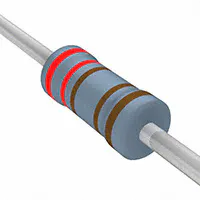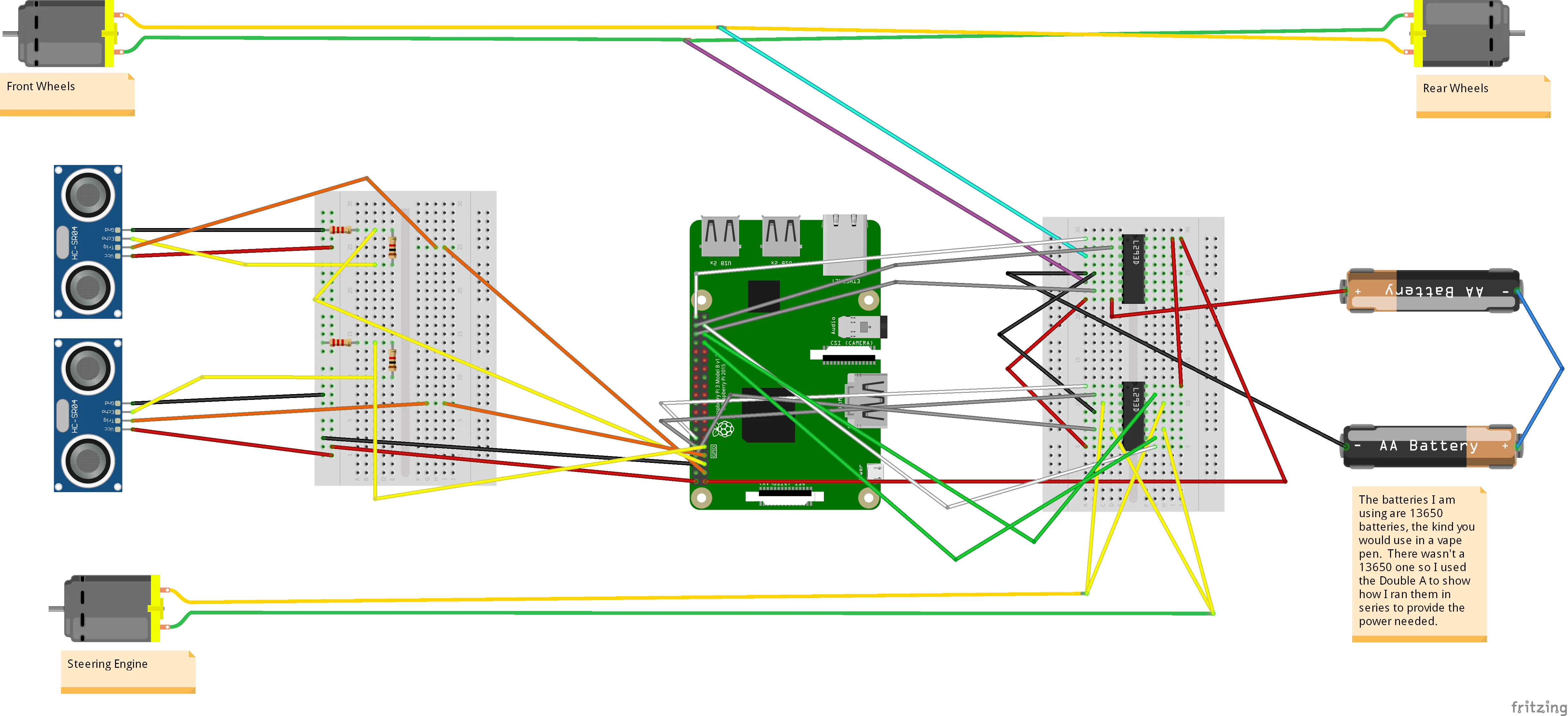# Raspberry Pi Autonomous RC Car

My first attempt at building an autonomous RC car with a Raspberry Pi 3.

BeginnerWork in progress10 hours14,194## Things used in this project

### Hardware components

 Monster Rockslide RC car
×1Raspberry Pi 3 Model B
×1Texas Instruments Dual H-Bridge motor drivers L293D
×2
 18650 Batteries
×1×2Jumper wires (generic)
×1Ultrasonic Sensor - HC-SR04 (Generic)
×2Resistor 1k ohm
×2Resistor 2.21k ohm
×2
 18650 Battery Holders
×1

### Software apps and online servicesRaspberry Pi Raspbian

## Schematics

### The component layout## Code

### First Try code

Python
If anyone has suggestions on how to make this better, I welcome any and all comments
```import RPi.GPIO as GPIO
import time

GPIO.setmode(GPIO.BOARD)

turnlefta =   8
turnleftb =   10
turnleftenable =   12

turnrighta = 33
turnrightb = 35
turnrightenable = 37

forwarda = 36
forwardb = 38
forwardenable = 40

trigger = 3
echo = 5

trigger1 = 7
echo1 = 11

GPIO.setup(trigger, GPIO.OUT)
GPIO.setup(echo, GPIO.IN)
GPIO.setup(trigger1,GPIO.OUT)
GPIO.setup(echo1, GPIO.OUT)
GPIO.setup(turnlefta, GPIO.OUT)
GPIO.setup(turnleftb, GPIO.OUT)
GPIO.setup(turnleftenable, GPIO.OUT)
GPIO.setup(turnrighta, GPIO.OUT)
GPIO.setup(turnrightb, GPIO.OUT)
GPIO.setup(turnrightenable, GPIO.OUT)
GPIO.setup(forwarda, GPIO.OUT)
GPIO.setup(forwardb, GPIO.OUT)
GPIO.setup(forwardenable, GPIO.OUT)
pwm=GPIO.PWM(40,100)
turn = GPIO.PWM(37,100)

J = True

while J:
GPIO.output(trigger,False)
GPIO.output(trigger1,False)
time.sleep(.5)

GPIO.output(trigger,True)
time.sleep(0.00001)
GPIO.output(trigger, False)

GPIO.output(trigger1,True)
time.sleep(0.00001)
GPIO.output(trigger1,False)

while GPIO.input(echo)==0:# and GPIO.input(echo1) == 0:
pulse_start = time.time()
#pulse1 = time.time()
while GPIO.input(echo)==1:# and GPIO.input(echo1) == 1:
pulse_end = time.time()
#pulse1end = time.time()

pulse_duration = pulse_end - pulse_start
#pulse1duration = pulse1end - pulse1
distance = pulse_duration * 17150
distance = round(distance, 2)
#distance1 = pulse1duration * 17150
#distance1 = round(distance1, 2)

if distance > 100:
pwm.start(0)
GPIO.output(36,True)
GPIO.output(38, False)
pwm.ChangeDutyCycle(30)
GPIO.output(40, True)
print("distance is ", distance)
elif distance >=75 <= 99:
pwm.ChangeDutyCycle(40)
GPIO.output(turnrighta, False)
GPIO.output(turnrightb, True)
turn.ChangeDutyCycle(80)
GPIO.output(turnrightenable,True)
time.sleep(1)
GPIO.output(turnrightenable,False)
GPIO.output(turnrighta, False)
GPIO.output(turnrightb, False)
print("turn distance ", distance)
elif distance >=30 <=74:
GPIO.output(36, False)
GPIO.output(38,False)
GPIO.output(40, False)
time.sleep(3)
GPIO.output(36, False)
GPIO.output(38, True)
pwm.ChangeDutyCycle(50)
GPIO.output(40, True)
GPIO.output(turnlefta, True)
GPIO.output(turnleftb, False)
GPIO.output(turnleftenable, True)
time.sleep(1)
GPIO.output(36,False)
GPIO.output(38,True)
GPIO.output(40,False)
GPIO.output(turnlefta, False)
GPIO.output(turnleftb, False)
GPIO.output(turnleftenable, False)
print("reverse distance ", distance)
elif distance <= 29:
GPIO.output(36, False)
GPIO.output(38,False)
GPIO.output(40, False)
GPIO.output(turnleftenable, False)
GPIO.output(turnrightenable, False)
time.sleep(1)
J = False
print("Program has Exited")

# if distance > 30 and distance < 300:

#    pwm.start(0)

#   GPIO.output(36, True)
#  GPIO.output(38, False)
# pwm.ChangeDutyCycle(50)

#      GPIO.output(40, True)

# if distance > 20 and distance < 50:
#    pwm.ChangeDutyCycle(30)

#  if distance < 10:
#     GPIO.output(40, False)
#    time.sleep(6)

#   GPIO.output(36,False)
#  GPIO.output(38, True)
# pwm.ChangeDutyCycle(50)
#GPIO.output(40,True)

#       time.sleep(3)

#      GPIO.output(40,False)
print("the current distance is:", distance)
GPIO.Cleanup

```

## Credits

### SG Tidwell

1 project • 2 followers
SQL slinging code monkey by day, guitar playing gamer, tinker by night.